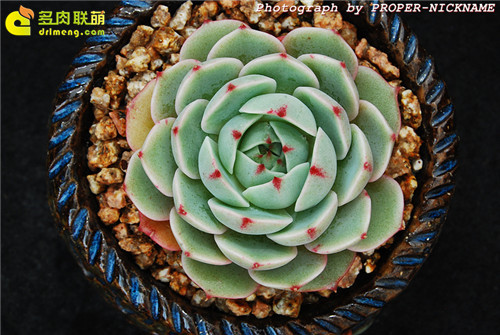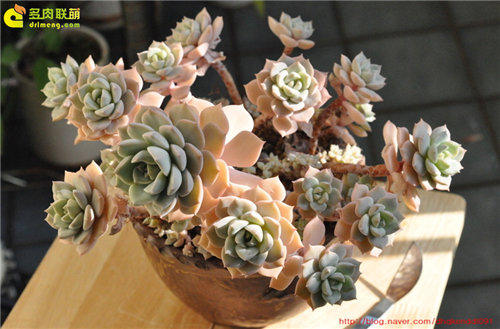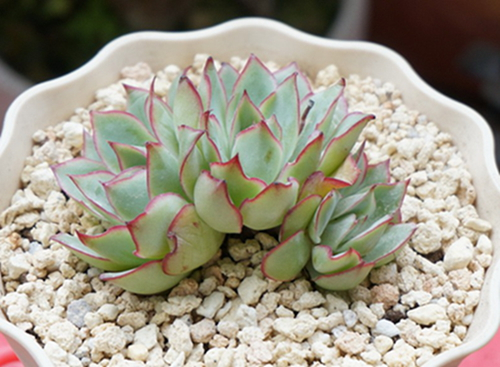25

一些景天的品种的杂交公式

一些景天的品种的杂交公式，有些在你看来平平无奇的普货，它们的父母可是大有来头，譬如白牡丹的母本是静夜，这也完美了解释了为什么他们动不动就黑腐。虽然是杂交公式，但不代表你选好父本母本就能泡制出同样的杂交品种，具体的来说就是虽然杂交公式是这样的静夜×乙女心=柳叶莲华，但你真拿静夜和乙女心杂交了真不一定出柳叶莲华。因为一个品种的选育往往要持续到第三代才能定性，你手里的柳叶莲华并不是简单的静夜×乙女心直接杂交出来的，而是杂交出来的品种————>再继续选育————>然后这个品种再繁殖————>才出来的柳叶莲华。
以下杂交公式为木兰君整理的，一般来说，母本在前，父本在后。
静夜×大和锦=苯巴蒂斯静夜×胧月=白牡丹静夜×花月夜=月光女神静夜×丽娜莲=露娜莲
静夜X鲁式=雪域
静夜×特玉莲=爱尔兰薄荷
静夜×花司=戴伦
静夜×锦司晃=玫瑰莲
静夜×粉蔓=船长甘草
静夜×月影=静月
静夜×吉娃娃=Tippy
静夜×乙女心=柳叶莲华
静夜×多茎莲=红化妆
静夜×黑爪=红爪
黑爪X雪莲=比安特
大和锦×桃之卵=葡萄
大和锦×静夜=法比奥拉
大和锦×E."Big Red"=央金
大和锦（杂）×晚霞=赫拉
姬莲×蓝石莲=蓝姬莲
玉蝶×姬莲=娜娜胡可
黑爪×姬莲=野蔷薇精灵
小和锦×姬莲=皇冠
广寒宫×沙维娜=晨光
广寒宫×沙维娜=晨星
广寒宫×沙维娜=晚霞
广寒宫×鲁氏=多明戈
广寒宫×鲁氏=赫斯塔
广寒宫×多明戈=星期天
广寒宫×皮氏=蓝鸟
广寒宫×星美人=霜之朝
沙维娜×广寒宫=Pinky
雪莲X原始卡罗拉=芙蓉雪莲
原始卡罗拉X雪莲=梦露
雪莲×特玉莲=特玉雪莲
古紫×沙维娜=黑王子
月影×粉彩莲=紫珍珠
月影×静夜=月静
花月夜×厚叶月影=月亮仙子
花月夜×大和锦=猎户座
花月夜 X 霜之鹤=花之鹤
锦晃星×锦司晃=白闪冠
特玉莲X黛比=丘比特
胧月 x 乙女心=秋丽
胧月 x 冬云系如贝拉=厚叶旭鹤
胧月 x 珊瑚珠=姬胧月
雪莲 X 霜之鹤=白凤
广寒宫 x鲁氏 =赫斯塔
广寒宫 x 刚叶莲=参宿
皮氏 x 刚叶莲=苏珊塔
钢叶莲x 雪莲=薄荷

★微信搜索公众号id drlmeng，即可关注联萌，更多互动★。

最后编辑于：2017/8/4作者：联萌小编